## How to find the most frequent text with criteria in Excel?

This article will talk about how to find the most occurred text based on the specific criteria from a range of cells in Excel. At the same time, I will also introduce the formula for extracting the most frequently occurring text in a column.

 Find the most frequent text Find the most frequent text based on criteria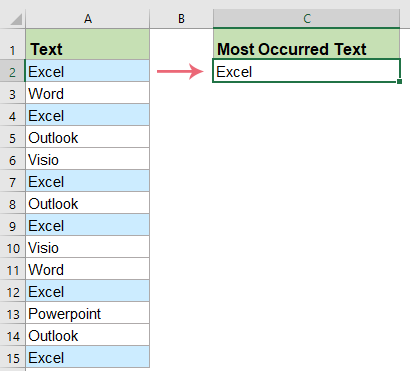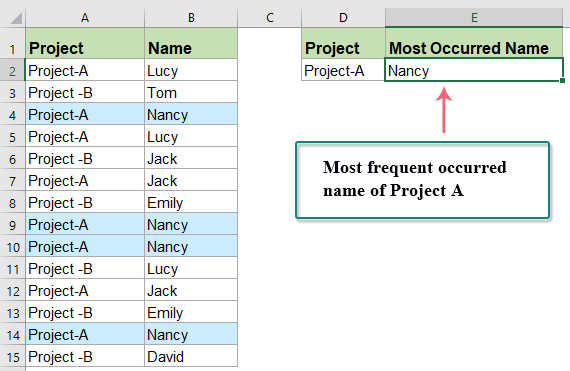#### Find the most frequent occurring text in a column with array formula

If you just want to find and extract the most occurred text from a list of cells, please apple the below formula:

The generic formula syntax is:

=INDEX(range, MODE(MATCH(range, range, 0 )))
• range: is the list of cells that you want to find the most frequent occurring text.

1. Enter or copy this formula into a blank cell where you want to output the result:

=INDEX(A2:A15,MODE(MATCH(A2:A15,A2:A15,0)))
• Tips: In this formula:
• A2:A15: is the data list that you want to find the greatest number of times a text occurs.

2. And then, press Ctrl + Shift + Enter keys simultaneously, and you will get the correct result as below screenshot shown: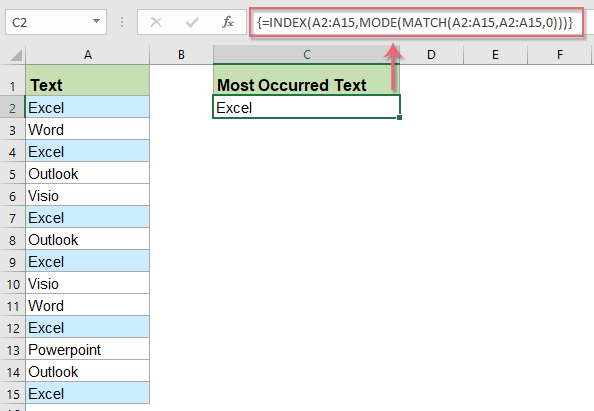#### Find the most frequent occurring text based on criteria with array formulas

Sometimes, you may need to find the most frequent occurring text based on a specific condition, for example, you want to find the most occurred name which Project is Project A as below screenshot shown: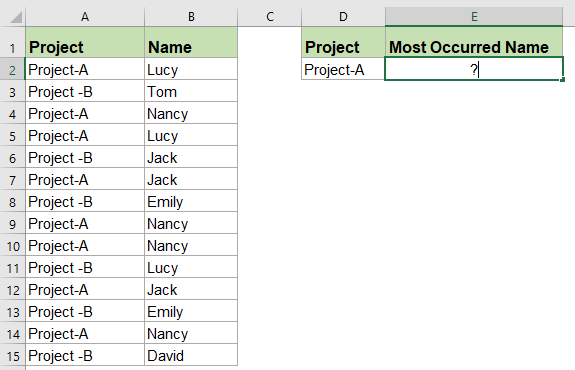The generic formula syntax is:

=INDEX(range1,MODE(IF(range2=criteria, MATCH(rang1,range1,0))))
• range1: is the range of cells that you want to find the most frequent occurring text.
• range2=criteria: is the range of cells contain the specific criteria that you want to find name based on.

1. Please enter or copy the below formula into a blank cell:

=INDEX(\$B\$2:\$B\$15,MODE(IF(\$A\$2:\$A\$15=D2,MATCH(\$B\$2:\$B\$15,\$B\$2:\$B\$15,0))))
• Tips: In this formula:
• B2:B15: is the data list that you want to find the greatest number of times a name occurs.
• A2:A15=D2: is the range of cells contain the specific criteria that you want to find text based on.

2. And then, press Ctrl + Shift + Enter keys simultaneously, the most frequent occurring name of Project A has been extracted, see screenshot: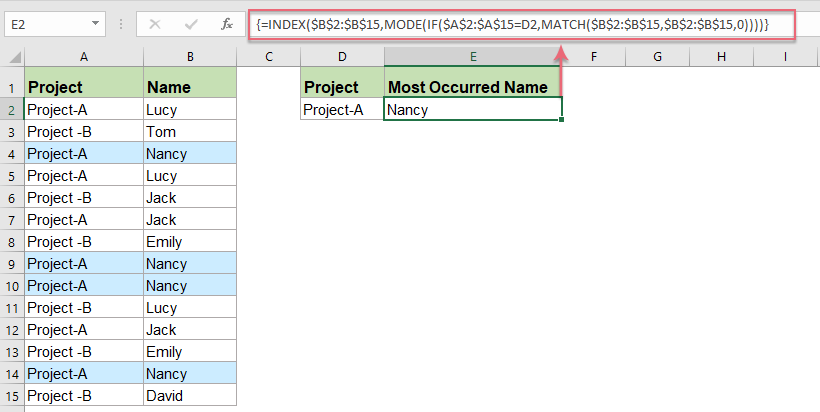#### Find the most frequent occurring text between two given dates with array formula

This section is going to talk about how to find the most common text which is between two given dates. For instance, to find the most frequent name based on the dates which is between 6/28/2019 and 7/5/2019, the following array formula may help you:

1. Please apply the below formula into a blank cell:

=INDEX(\$B\$2:\$B\$15, MATCH(MODE.SNGL(IF((\$A\$2:\$A\$15<=\$E\$2)*(\$A\$2:\$A\$15>=\$D\$2), COUNTIF(\$B\$2:\$B\$15, "<"&\$B\$2:\$B\$15), "")), COUNTIF(\$B\$2:\$B\$15, "<"&\$B\$2:\$B\$15),0))
• Tips: In this formula:
• B2:B15: is the data list that you want to find the greatest number of times a name occurs.
• A2:A15<=E2: is the range of dates that less than or equal to a specific date that you want to find the name based on.
• A2:A15>=D2: is the range of dates that greater than or equal to a specific date that you want to find the name based on.

2. And then, press Ctrl + Shift + Enter keys together, the most frequent occurring name between the specific two dates has been extracted, see screenshot: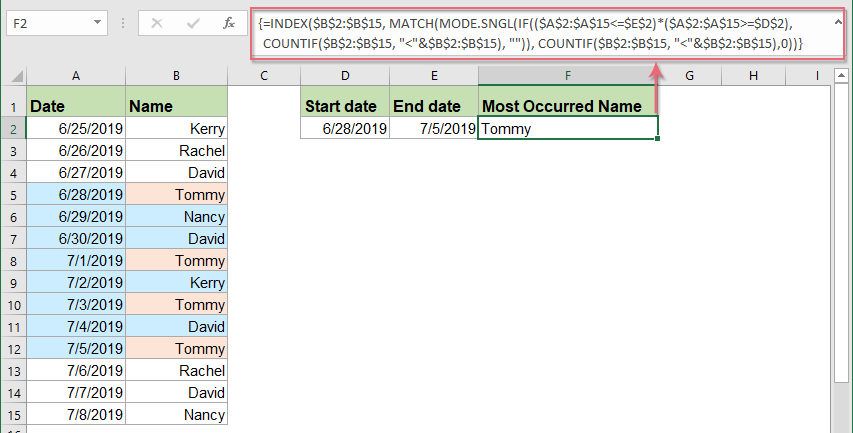#### More relative most frequent text articles:

• Find The Second Most Common/Frequent Number Or Text In Excel
• We can apply the MODE function to find out the most frequent number from a range at ease in Excel. However, how about finding out the second most frequent number from a column? And what if finding out the second most common text value from a column? Here we get some workarounds for you.
• Find Most Common Value(Number Or Text String) From A List In Excel
• Supposing you have a list of names which contain some duplicates, and now, you want to extract the value that appears the most frequently. The direct way is to count the data one by one from the list to get the result, but if there are thousands of names in the column, this way will be troublesome and time-consuming. The following tutorial will introduce you some tricks to solve this task quickly and conveniently.
• Sort Data By The Most Frequent Value In Excel
• Supposing you have a long list of data in your worksheet, and now you would like to sort this list by the frequency of which each word occurs. That is to say, the most common value (for example, occurs four times in the column) is listed first, and then be followed by the words which occur three times, twice and once as following screenshots shown. How could you solve this task in Excel?

## The Best Office Productivity Tools

### Kutools for Excel Solves Most of Your Problems, and Increases Your Productivity by 80%

• Super Formula Bar (easily edit multiple lines of text and formula); Reading Layout (easily read and edit large numbers of cells); Paste to Filtered Range...
• Merge Cells/Rows/Columns and Keeping Data; Split Cells Content; Combine Duplicate Rows and Sum/Average... Prevent Duplicate Cells; Compare Ranges...
• Select Duplicate or Unique Rows; Select Blank Rows (all cells are empty); Super Find and Fuzzy Find in Many Workbooks; Random Select...
• Exact Copy Multiple Cells without changing formula reference; Auto Create References to Multiple Sheets; Insert Bullets, Check Boxes and more...
• Favorite and Quickly Insert Formulas, Ranges, Charts and Pictures; Encrypt Cells with password; Create Mailing List and send emails...
• Extract Text, Add Text, Remove by Position, Remove Space; Create and Print Paging Subtotals; Convert Between Cells Content and Comments...
• Super Filter (save and apply filter schemes to other sheets); Advanced Sort by month/week/day, frequency and more; Special Filter by bold, italic...
• Combine Workbooks and WorkSheets; Merge Tables based on key columns; Split Data into Multiple Sheets; Batch Convert xls, xlsx and PDF...
• Pivot Table Grouping by week number, day of week and more... Show Unlocked, Locked Cells by different colors; Highlight Cells That Have Formula/Name...### Office Tab - brings tabbed interface to Office, and make your work much easier

• Enable tabbed editing and reading in Word, Excel, PowerPoint, Publisher, Access, Visio and Project.
• Open and create multiple documents in new tabs of the same window, rather than in new windows.
• Increases your productivity by 50%, and reduces hundreds of mouse clicks for you every day!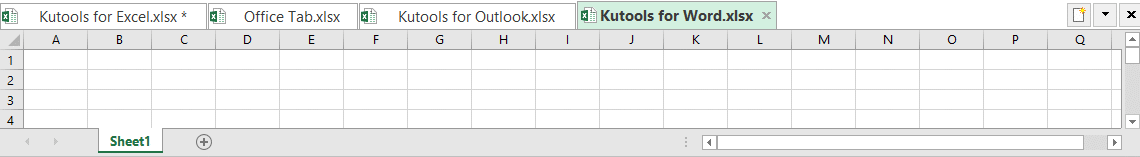No ratings yet. Be the first to rate!
This comment was minimized by the moderator on the site
Hello, thank you for your help.
I've used the "Find the most frequent occurring text based on criteria with array formulas" which works well for the 14 rows of data in your example however, when I increase the rows (and the formula values to match with CTRL+SHIFT+ENTER) it is still only searching the first 14 rows and returning "N/A" searching for anything past row 14.

Thank you
This comment was minimized by the moderator on the site
Hallo, eine wunderbare Lösung, funktioniert auch super - nur in meinem speziellen Fall noch nicht ganz: wenn im Suchbereich auch Leerzellen sind, also z.B. hier bei "Name" im Bereich "Finden Sie den am häufigsten vorkommenden Text anhand von Kriterien mit Array-Formeln", da sind bei mir auch leere Zellen dabei. Schon kommt überall nur noch "#NV". Gibt es da eine Lösung, um trotzdem noch den häufigsten (Text)-Inhalt zu finden? Vielen Dank!
This comment was minimized by the moderator on the site
...bzw. mit der Formel aus #41657 kommt kein #NV mehr, dafür aber auch nur manchmal ein Ergebnis, was nicht Null ist. Manchmal kommt der richtige Wert, häufig halt nur "0". ..:Danke :-)
This comment was minimized by the moderator on the site
Bagaimana jika range yang kita cari terdapat kolom kosongnya? misal kita buat rumus paten raport, kita ingin mencari akhlak umumnya siswa, kita ambil jangkauan terjauh, misal dalam 1 kelas, jumlah maksimal anak 60. pada suatu kelas lain jumlah siswa cuma 50, sehingga kolom 51 sd 60 kosong. apakah rumus demikian masih valid? saya coba kok #N/A atau mohon pencerahannya!
This comment was minimized by the moderator on the site
Hi, This is great, what I found is that when there is tie or only one record the formula results in N/A. How do I get it to show the single result? this is when I want to find the most common value based on criteria.
This comment was minimized by the moderator on the site
Hello, Yeslam,

=IFERROR(INDEX(\$B\$2:\$B\$15,MODE(IF(\$A\$2:\$A\$15=D2,MATCH(\$B\$2:\$B\$15,\$B\$2:\$B\$15,0)))),INDEX(B2:B15,MATCH(D2,A2:A15,0)))

This comment was minimized by the moderator on the site
HEY! This is really helpful. I'm wondering if you could help me, with, if there is a tie, to pick one of the top values/strings. thanks!
This comment was minimized by the moderator on the site
This is really helpful. I'm just thinking how to do this with a third criteria for "Find the most frequent text based on criteria"? let say Inventory, Project, Name then get the most occured name.

Thank you so much!
This comment was minimized by the moderator on the site
Hello,
To apply the fromula for three criteria, please apply the below formula:
``=INDEX(\$A\$2:\$A\$12,MODE(IF(\$A\$2:\$B\$12=F2,IF(\$C\$2:\$C\$12=G2,IF(\$D\$2:\$D\$12=H2,MATCH(\$A\$2:\$A\$12,\$A\$2:\$A\$12,0))))))``

Tips：If you have more criteria, you just need to add the IF criteria as below:
``=INDEX(range,MODE(IF(range1=criteria1,IF(range2=criteria2,IF(range3=criteria3,... MATCH(rang,range,0))))))``

This comment was minimized by the moderator on the site
Halo gan,
bagaimana cara menemukan teks yang paling sering keluar KEDUA, KETIGA dan seterusnya?
Terima kasih :)
This comment was minimized by the moderator on the site
Hello, Dodik,
To solve your problem, please use the following formulas: (Please remember to press Ctrl + Shift + Enter keys together to get the result)

Find the second most common value:
``=INDEX(A1:A20,MODE(IF((A1:A20<>"")*(A1:A20<>INDEX(A1:A20,MODE(IF(A1:A20<>"",MATCH(A1:A20,A1:A20,0))))),MATCH(A1:A20,A1:A20,0))))``

Find the third most common value:
``=INDEX(A1:A20,MODE(IF(((A1:A20<>"")*(A1:A20<>INDEX(A1:A20,MODE(IF(A1:A20<>"",MATCH(A1:A20,A1:A20,0)))))*(A1:A20<>INDEX(A1:A20,MODE(IF((A1:A20<>"")*(A1:A20<>INDEX(A1:A20,MODE(IF(A1:A20<>"",MATCH(A1:A20,A1:A20,0))))),MATCH(A1:A20,A1:A20,0)))))),MATCH(A1:A20,A1:A20,0))))``
This comment was minimized by the moderator on the site
Thank you for this information, but what happens if there is a tie? For the generic example, =INDEX(A2:A15,MODE(MATCH(A2:A15,A2:A15,0))), how would the formula be modified to output text indicating that a majority could not be determined?
This comment was minimized by the moderator on the site
Hello, Lauren

If there are more than one result, you can use the below formulas:
First, you can use this array formula: (Note: after inserting the formula, please press Ctrl + Shift + Enter keys together to get the first result.)
``=INDEX(\$A\$2:\$A\$10,MATCH(MIN(MODE(IF(NOT(COUNTIF(C\$1:C1,\$A\$2:\$A\$10)),(COUNTIF(\$A\$2:\$A\$10,"<"& \$A\$2:\$A\$10)+1)*{1,1}))),COUNTIF(\$A\$2:\$A\$10,"<"& \$A\$2:\$A\$10)+{1},0))``

With this formula, you can extract all data from the most occurred value to least common value.
And then, you should use the below formula to count the number of the occurred text:
``=COUNTIF(\$A\$2:\$A\$10, C2)``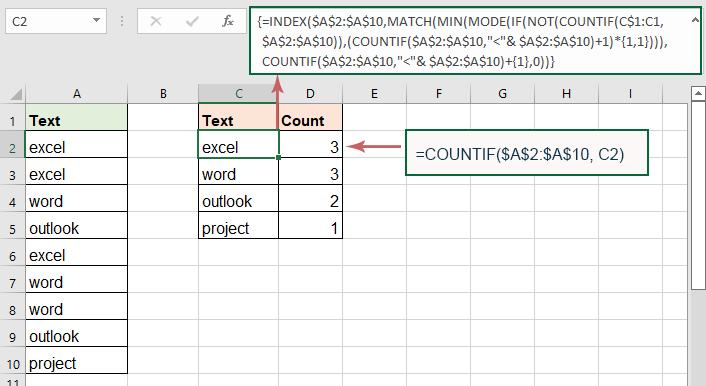This comment was minimized by the moderator on the site
Very helpful advice in this particular post! It’s the little changes that make the largest changes. Thanks for sharing!
This comment was minimized by the moderator on the site
Thank you for your post, I look for such article along time, today i find it finally. this post give me lots of advise it is very useful for me. website
This comment was minimized by the moderator on the site
Thanx for sharing the article, its really helpful.
This comment was minimized by the moderator on the site
Hello,